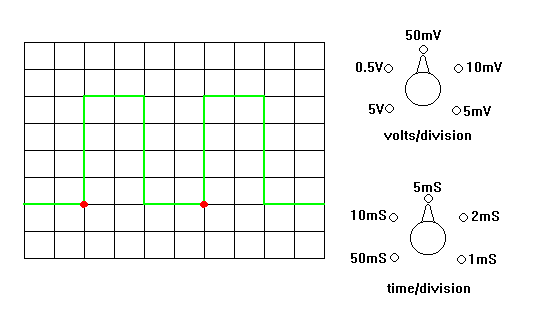Electronic Circuits and Tutorials
Tutorials
Dictionaries
Projects
Discover
Home > Electronics Tutorial > Test and Measurement > Using a Scope Tutorial
Test and Measurement in Electronics

# Using a Scope Tutorial

The controls on a scope can be divided into four groups.

(1) Housekeeping   (on/off, brightness, focus)

(2) Horizontal   (X position, X amplitude, time/division)

(3) Vertical   (Y position, Y amplitude, Invert, Volts/division)

(4) Trigger/Synchronization   (level, +/-, external, ac/dc/lf/hf, tvh/tvv)

Set any controls marked "calibrate" to the correct position.

Adjust the housekeeping controls to obtain a trace (display).

Adjust the vertical and horizontal controls to display a few cycles of a waveform.

Adjust the trigger/synch controls to make the display stationary.

It is best to display about two cycles and make them as large in height as possible.

Measure the amplitude and periodic time (time of one cycle). See below.

Calculate the frequency.In the diagram the waveform is 4 divisions high.

The volts/ division switch is set to 50 mV/division.

The amplitude is therefore 4 x 50 mV = 200 mV.

The width of one cycle (indicated between the two red dots) is 4 divisions.

The time/division switch is set to 5 mS/division.

The periodic time is 4 x 5 mS = 20 mS.

Note that one cycle is the time between the start of a waveform and the point where it starts to repeat itself.

Frequency can be calculated by dividing 1 second by the periodic time.

Note that if the periodic time is in mS then 1 second has to be expressed as 1000 mS.

1000mS/20mS = 50 Hz.

The pattern of squares is called a GRATICULE

Note: To report broken links or to submit your projects, tutorials please email to Webmaster